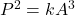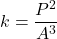## Kepler’s third law states that for all objects orbiting a given body, the cube of the semimajor axis (A) is proportional to the square of th

Question

Kepler’s third law states that for all objects orbiting a given body, the cube of the semimajor axis (A) is proportional to the square of the orbital period (P). For each of our planets orbiting the Sun, the relationship between the orbital period and semimajor axis can be represented by the equation P2 = kA3, where k is a constant value for all the planets. Rewrite this equation so that it solves for the constant of proportionality, k.

in progress 0
5 months 2021-09-04T07:46:49+00:00 1 Answers 12 views 0

## Answers ( )

1. Explanation:

Kepler’s third law states that for all objects orbiting a given body, the cube of the semimajor axis (A) is proportional to the square of the orbital period (P).

For each of our planets orbiting the Sun, the relationship between the orbital period and semimajor axis can be represented by the equation as:k is constant of proportionality

It is required to solve the above equation for k